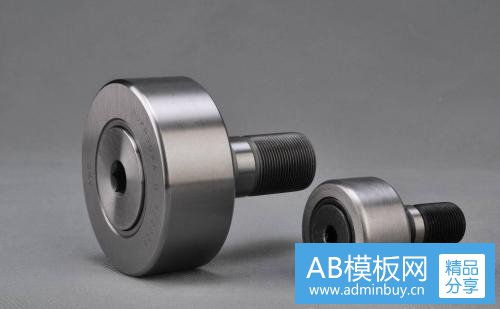# 滚动轴承的稳健化估计及性质M估计及性质
X;={x;(n)}, i=1,2,,m;n=1,2,.,N
(2-8)式中，X;为随机变量(Variable)时间序列，x;(n)为第 i次实验的第n个数据
i 为实验（experiment)序号，m为实验次数，n为数据（data)序号，N为数据个数。

Ai=x,(n),i=1,2,,m;n=1,2,,N

2()-1)”
(2-10)Q。
(1)=
N’
↑为目标函数自变量，x()为第i次实验的第n个数式中，Q(0)为1的目标函数。

20= ,入
i= ,,m;n= 12,.,
(2-11)式中，Q0为1的目标(cause)函数，p(x()()为目标函数估计函数, 1为目

p,(t)=t2, i=1,2,.,m
(2-12)式中，p()为第i次实验估计函数，I 为目标(cause)函数自变量，i 为实验序

Z(x(m)-1)
S
(1)=号
N，i= 12.,m;n=.2-,N
(2-13) 式中，S(0为目标(cause)函数，1为目标函数自变量，x(n)为第i

p,(t)=1, i=1,2,.,m
(2-14)

\$()= min之pp(x(n)-1,)， -2=2.,2-1.2,。
(2-15)式中，S0)为目标函数，1为目标函数自变量。
ln为x()数据的估计值，x(n)为第i次

M估计也可以用另外一种形式表示:
J(0)= ZJ,(x(n)-1.)=0,
i2=,,m;n=1,2,-,N
(2-16)式中，J(0为目标函数，↑为目标函数自变量，n为x
()数据的估计值，X)为第i次实验的第n个数据，为实验序号，m为实验次数，n为数据序号，N为数据个数。

1) Huber M估计
Huber M估计是在极小极大化以及Hampel这两个数据稳健化准则下的一种*优估计，其中Huber M的分布函数